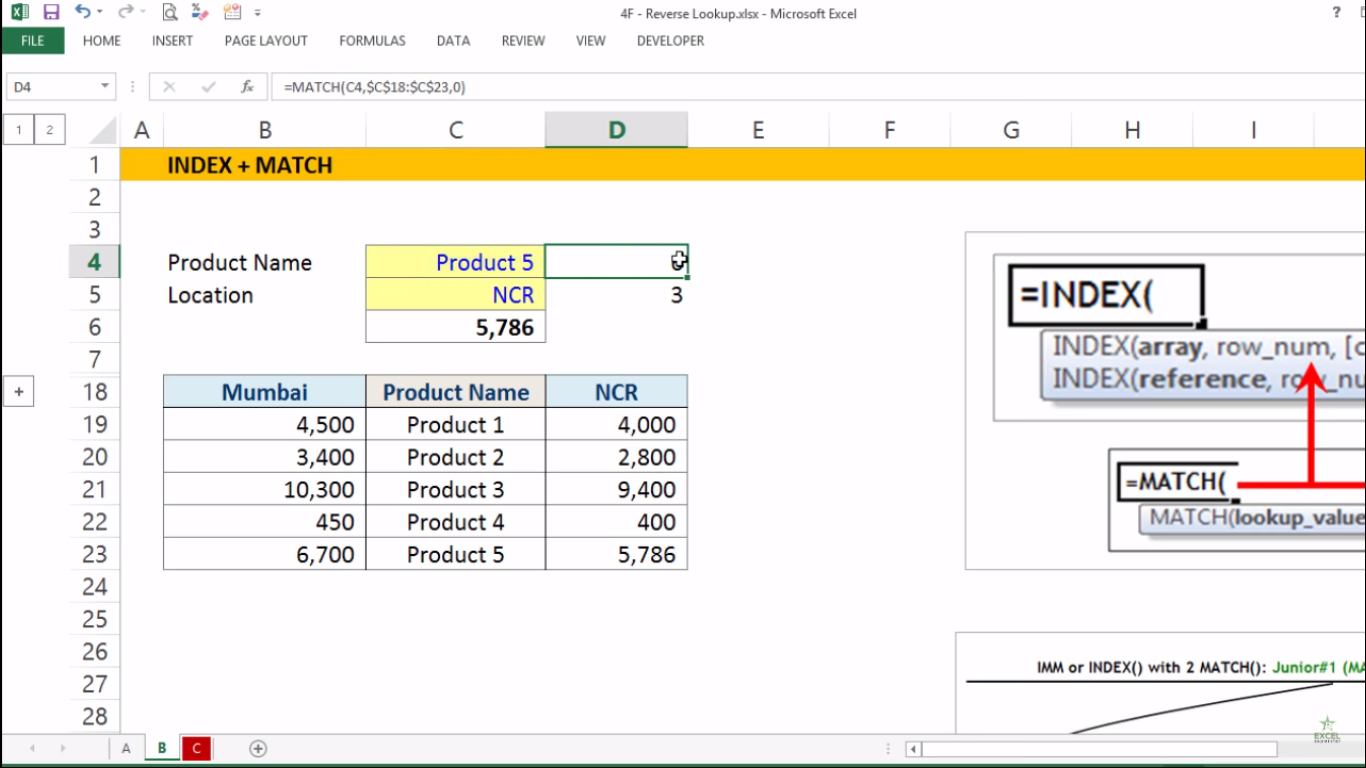# Advanced Excel Index + Match FormulaIn this tutorial we will learn the Index and Match formula in Advanced Excel with the help of an Example given below:

Example: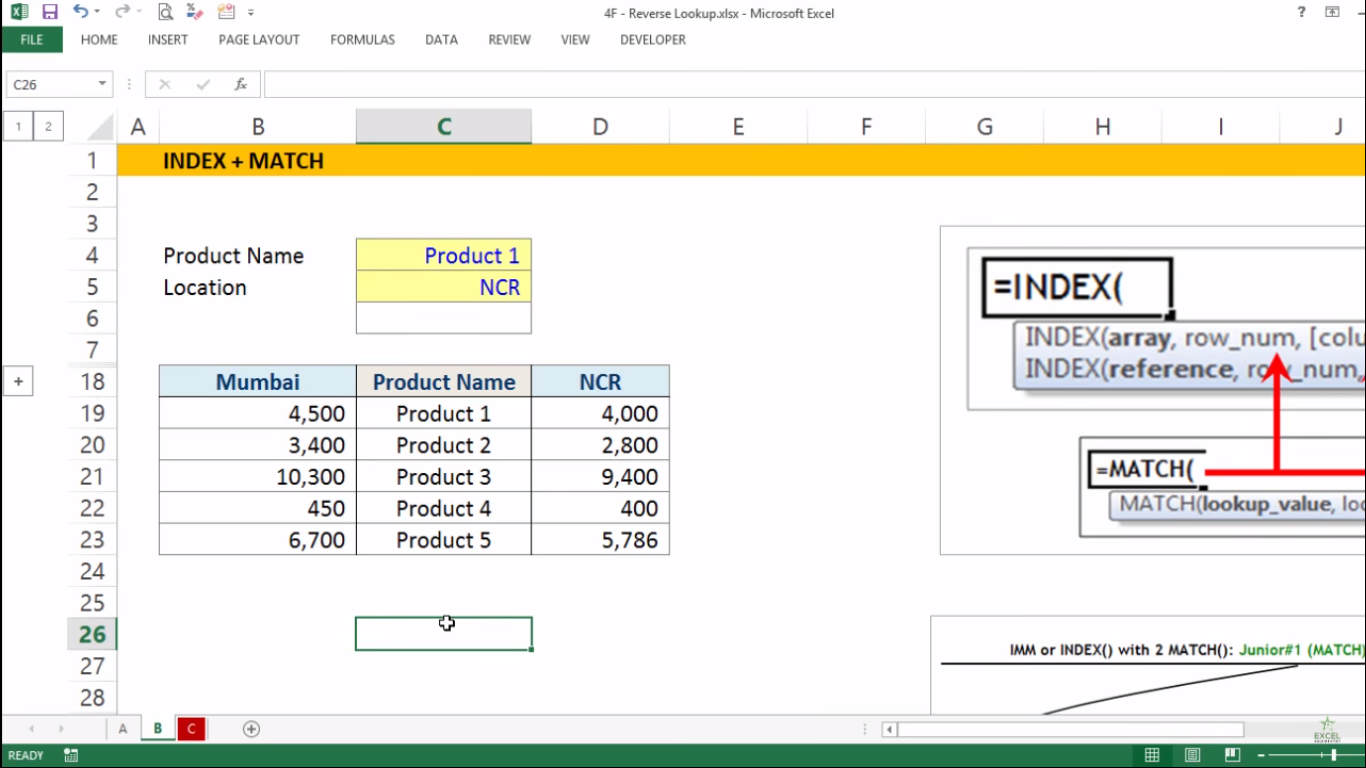I have a table that consists of Product Names and the prices of Mumbai and the NCR Region.

Now I want data where ID is in the Middle column then. My formula will be to pick the values from the left side of the data or the right-side data.

For Example:

If we choose the location as Mumbai and Product 1 as Product Name, we will pick Product 1 from Mumbai Location and fit into the blank cell to get the answer.
So to get the answer, we will be applying the INDEX and MATCH formula. Follow the steps given below to find out the answer.

1. Write =INDEX and press the tab keyNow there are 3 parameters (array, row_num, [column_num]).

The first parameter array means selecting the entire data inside the table

2. Choose the entire table and press F4 along with comma =INDEX(
\$B\$18:\$D\$23,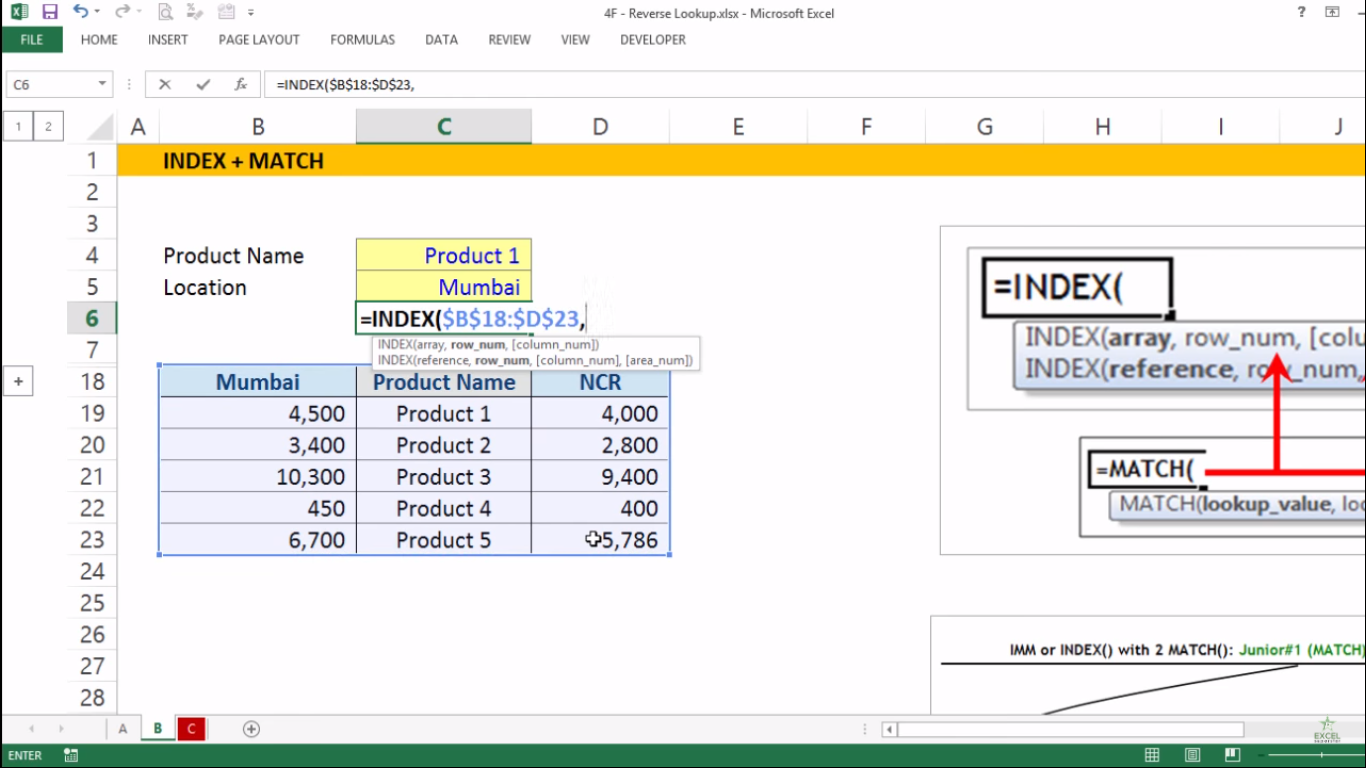The second parameter row_num means the row number in the a rray from which to return a value

3. So write 2 =INDEX( \$B\$18: \$D\$23,2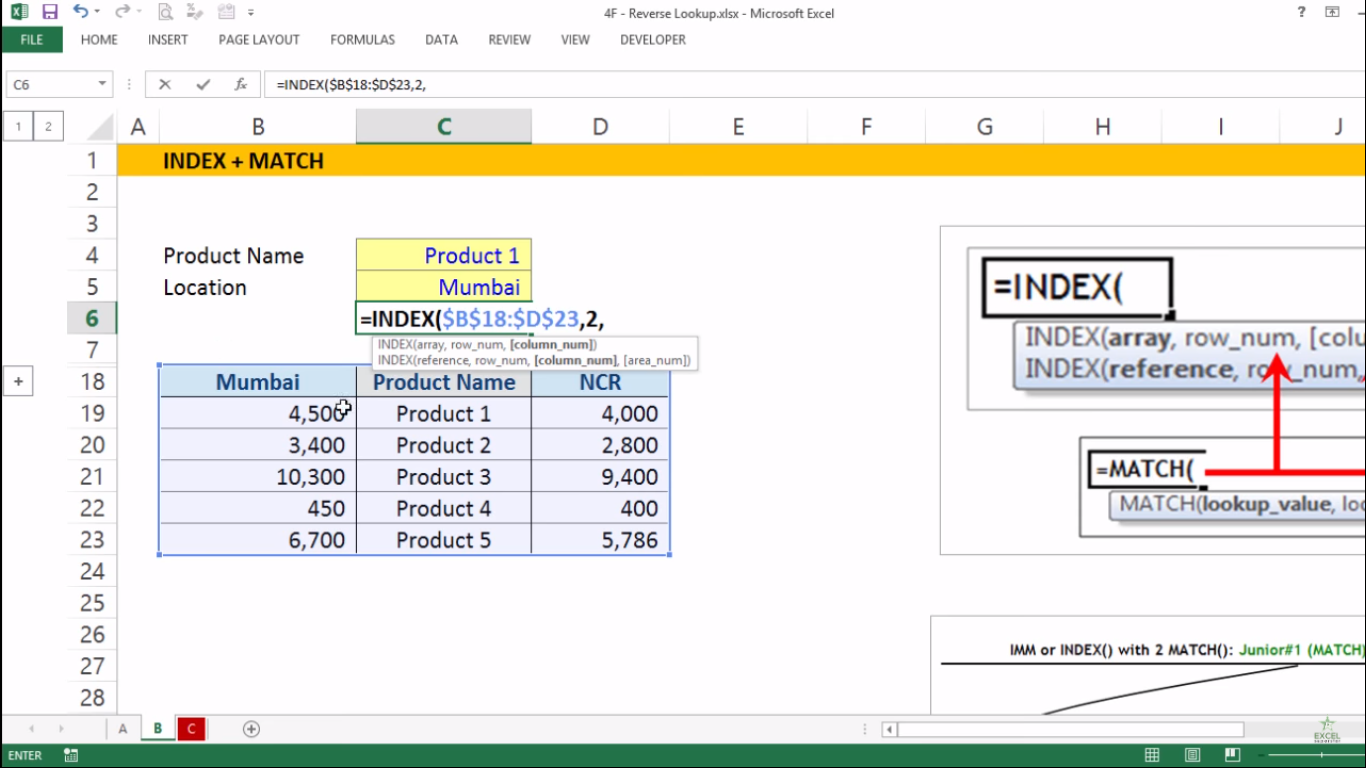Note – Here we are writing 2 because the table is talking about Product 1 which lies in the second Row

The second parameter [column_num] means the column number from which to return a value

4. So write 1 and close the bracket =INDEX( \$B\$18: \$D\$23,2,1)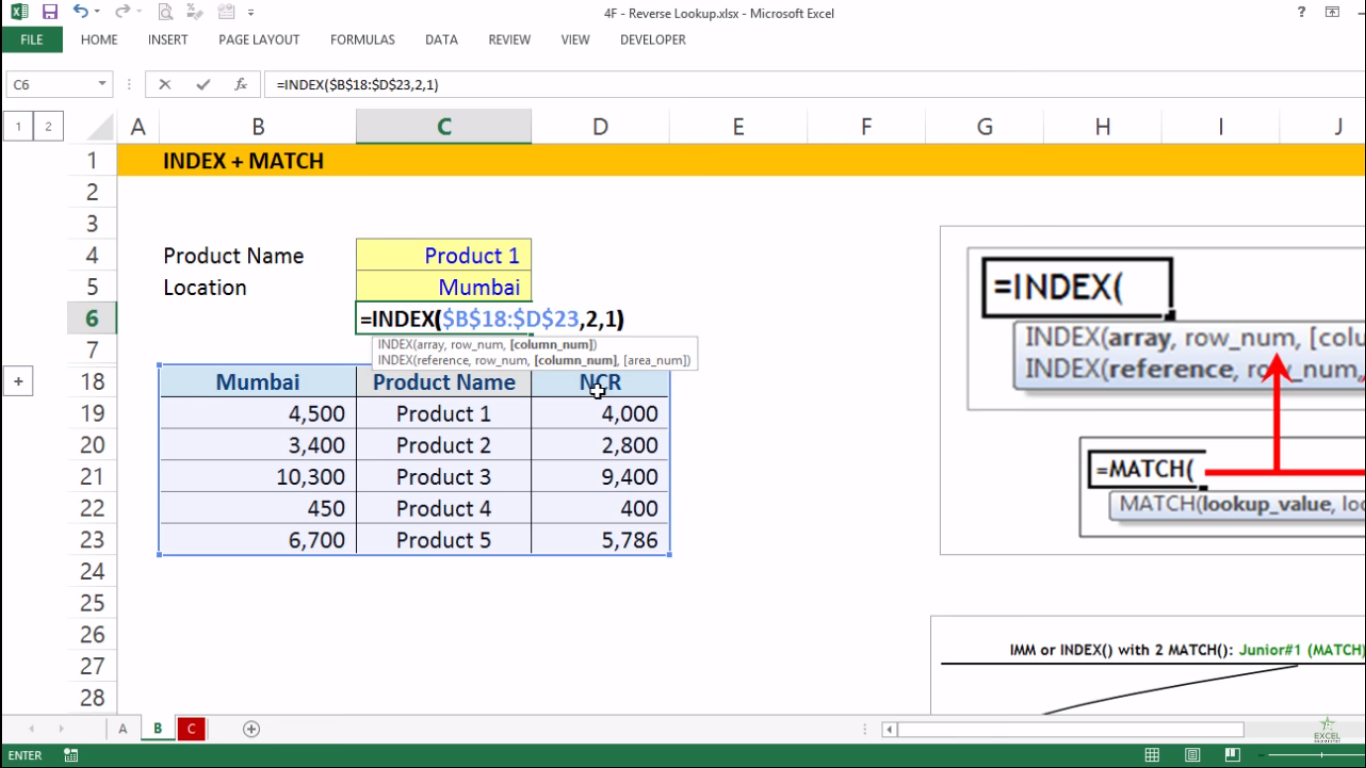5. Lastly, press Enter and you will get your answer as 4,500 which is the price of Product 1 for Mumbai Region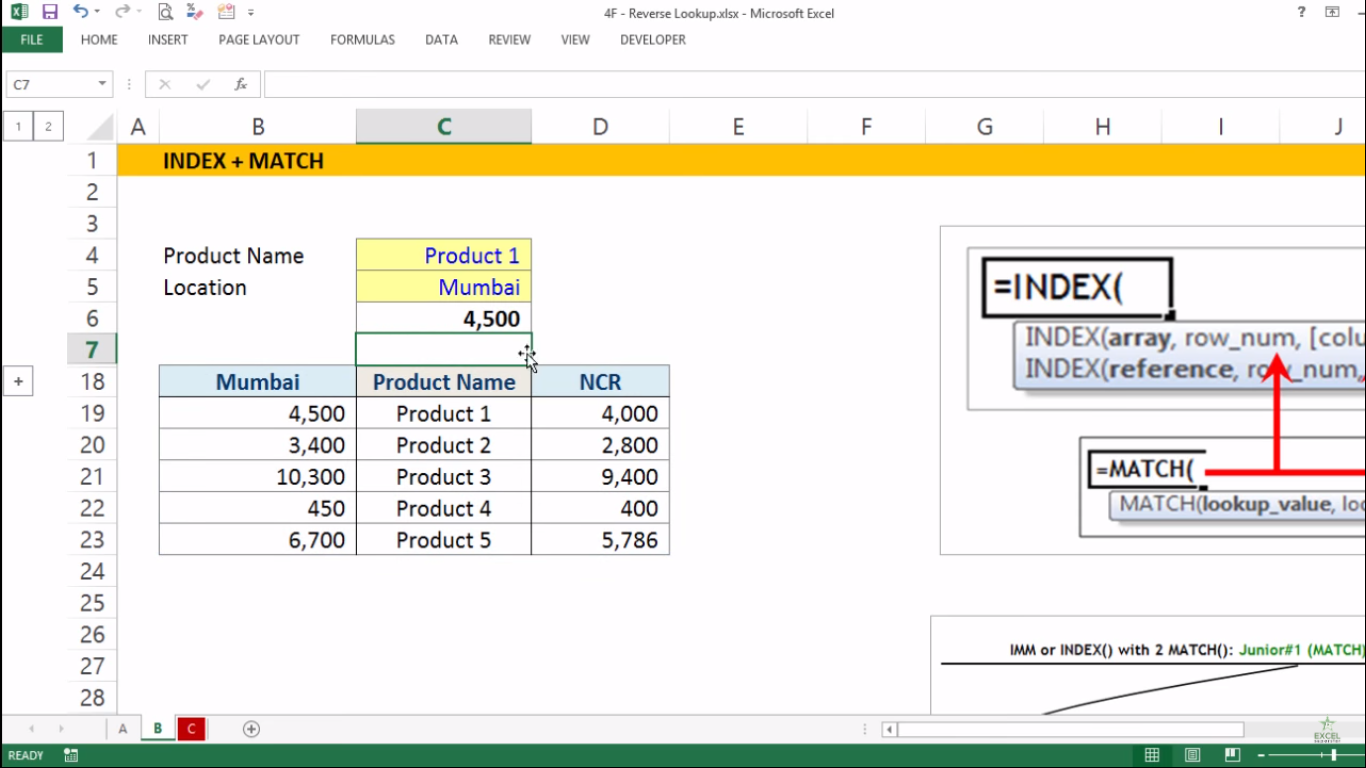But if I change the Product Name and Location to get the answer then the answer won’t change because the row_num and col_num inside the INDEX Formula is not changed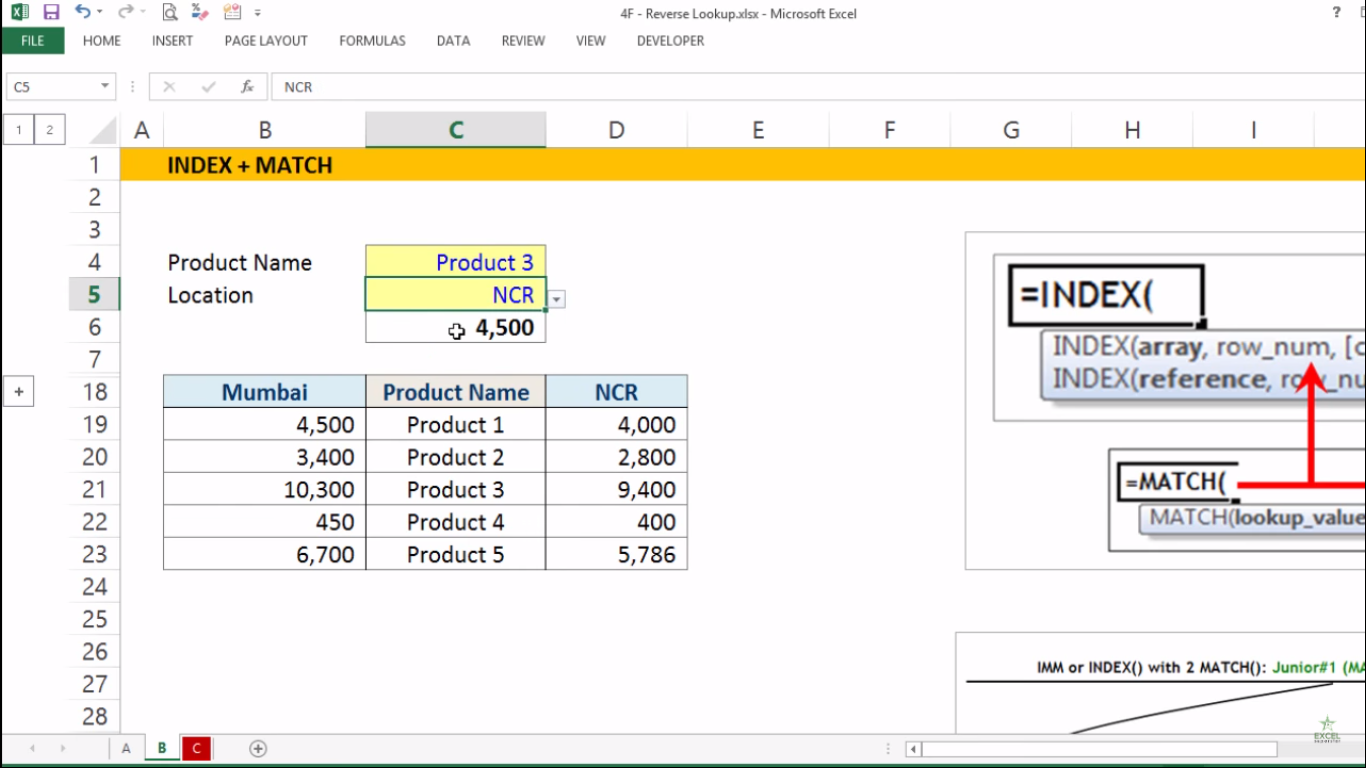So here we will be applying MATCH Formula inside the INDEX Formula to get the answer manually whenever we change the Location and Product Name in the given table

Note –
1. The row_num counting should always be from top to bottom
2. The col_num counting should always be from left to right

For Row_Num

1. Replace the row_num and write MATCH formula to reserve their seats MATCH()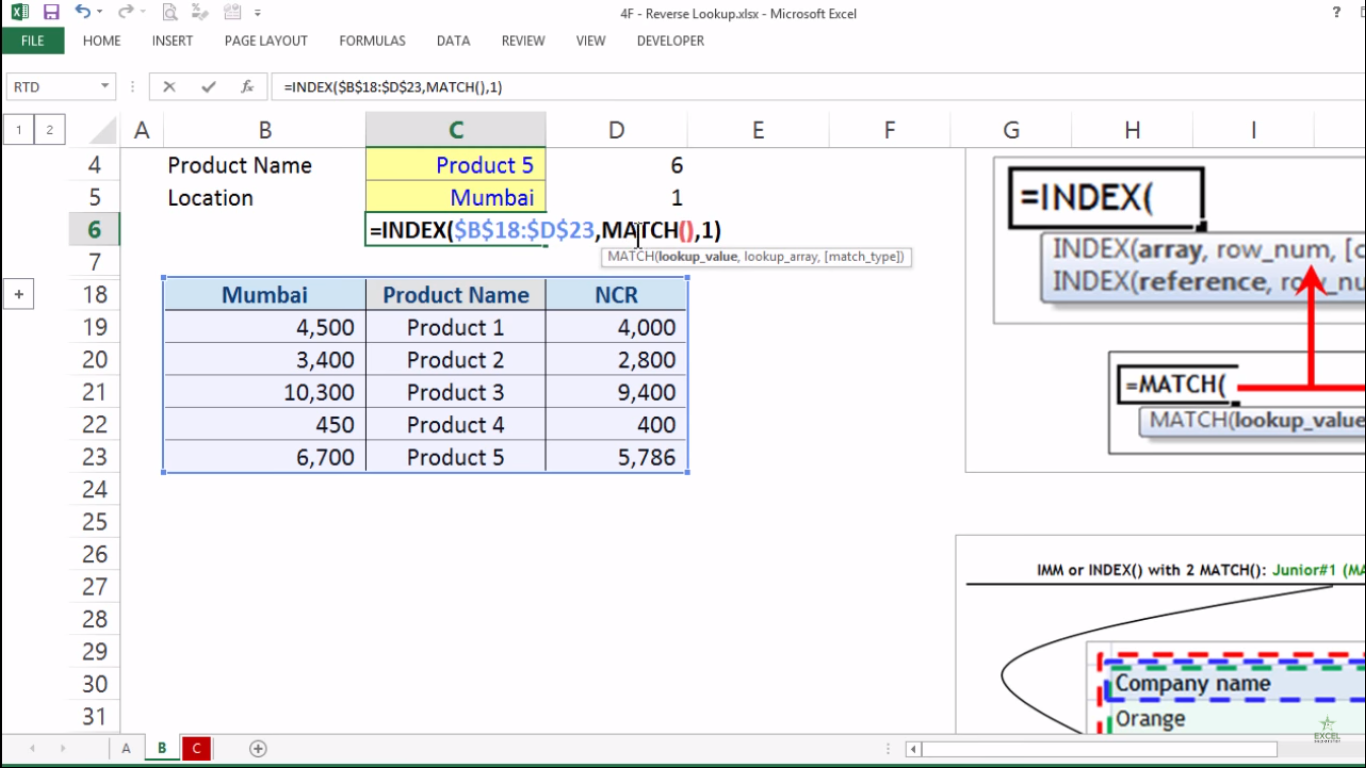Now inside the bracket there are 3 parameters (lookup_value, lookup_array, [match_type])

The first parameter lookup_value means the value to match in lookup_array.

2. Select the Product 5 cell =MATCH(C4,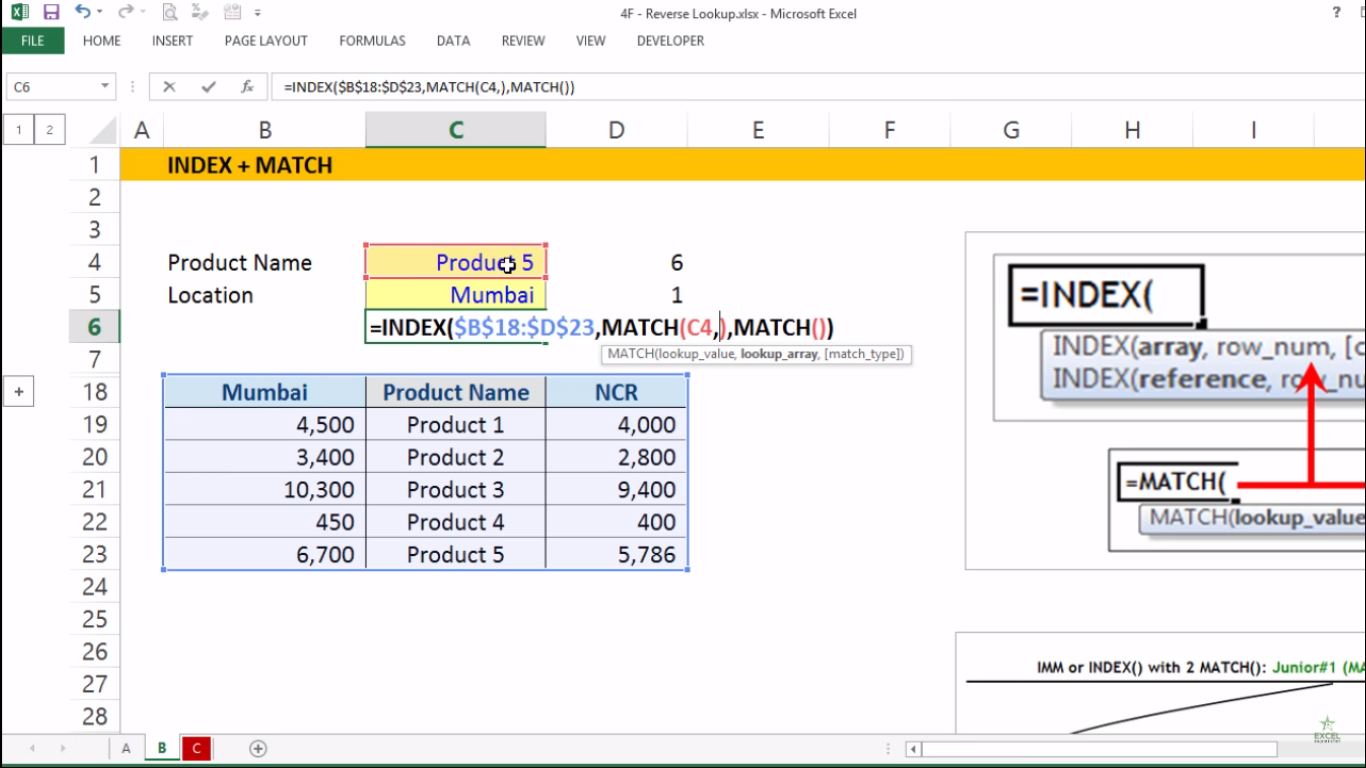The second parameter lookup_array means range of cells

3. Select the Product Name Column from top to bottom and press F4 =MATCH(C4,\$C\$18:\$C\$23,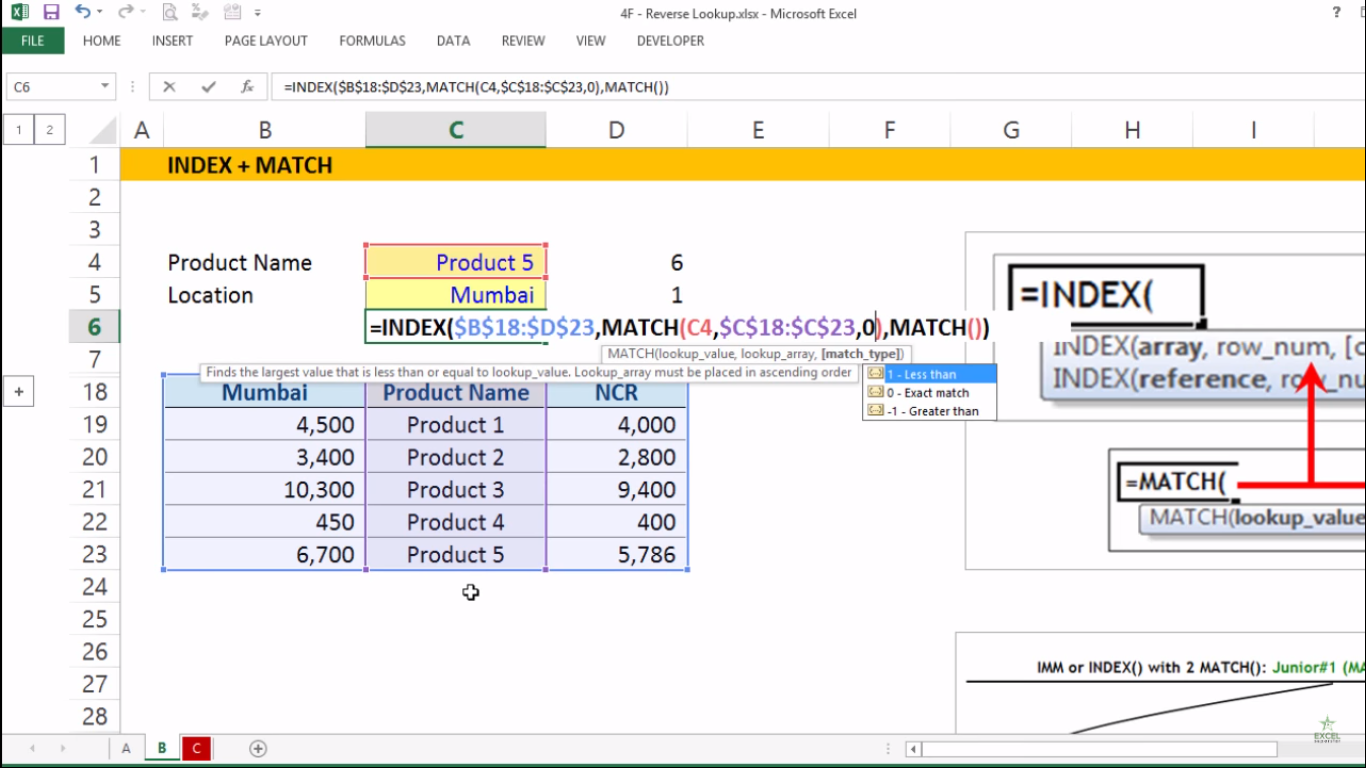The third parameter match_type means 1 =less than 0 = exact match, -1 = greater than

6. Write 0 as exact match and close the bracket =MATCH(C4,\$C\$18:\$C\$23,0)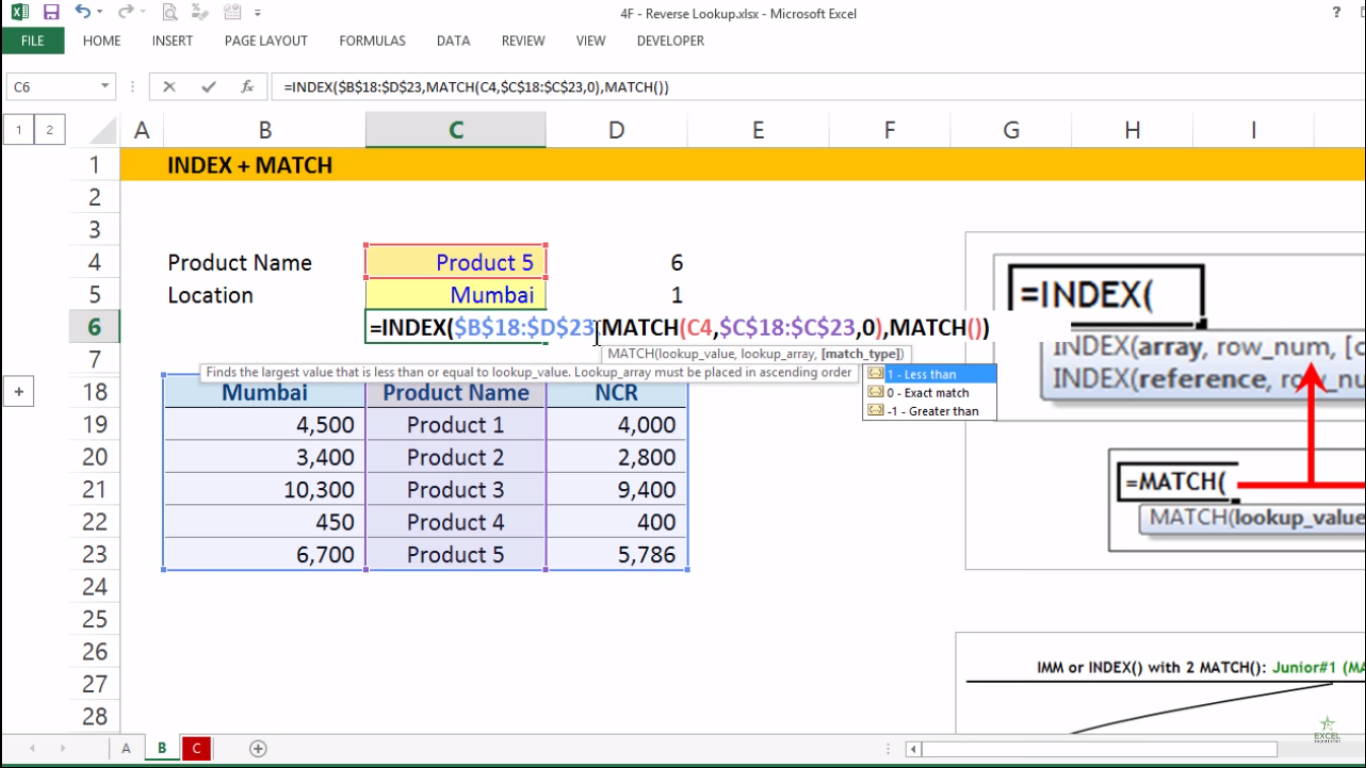For Col_num

1. Replace the col_num and write MATCH formula to reserve their seats MATCH()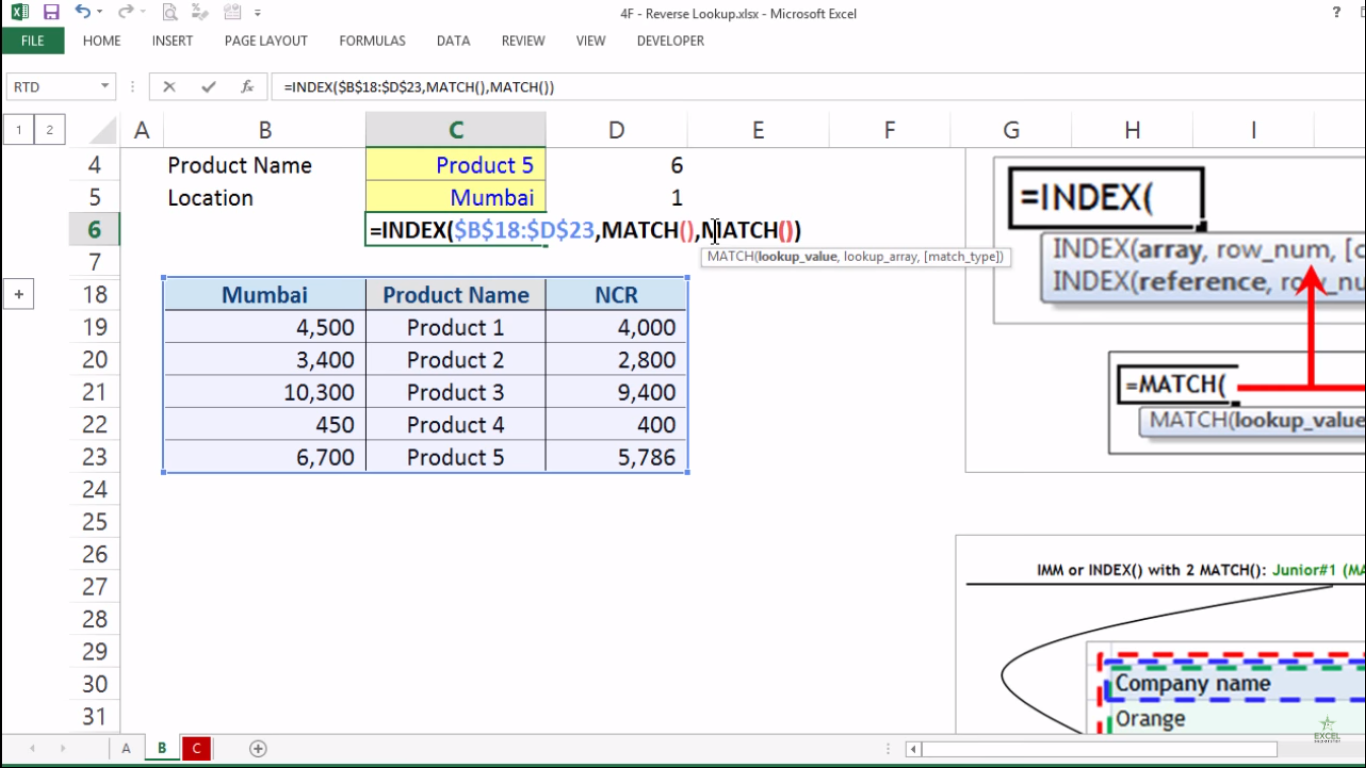Now inside the bracket there are 3 parameters (lookup_value, lookup_array, [match_type])

The first parameter lookup_value means the value to match in lookup_array.

2.  Select the Mumbai cell =MATCH(C5,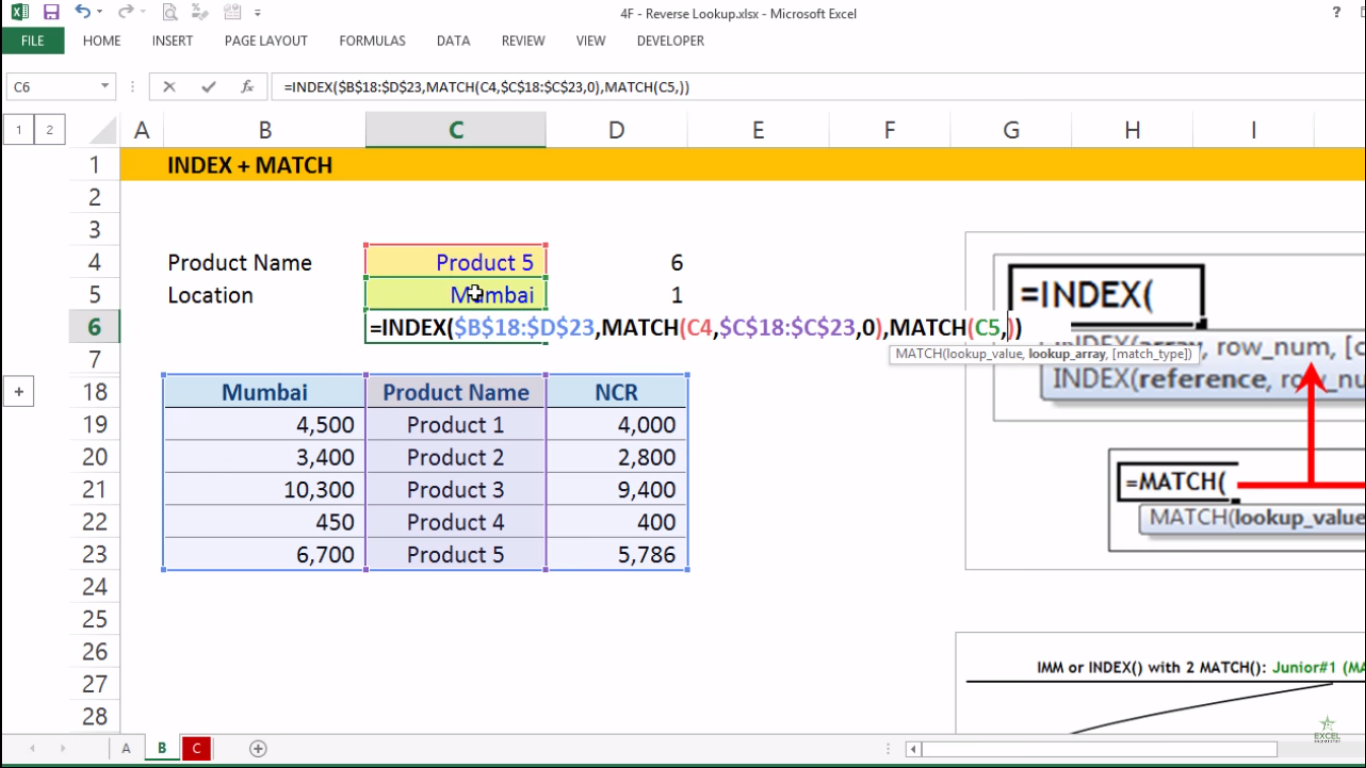The second parameter lookup_array means range of cells

3. Select the headers from left to right and press F4 =MATCH(C5,\$B\$18:\$D\$18,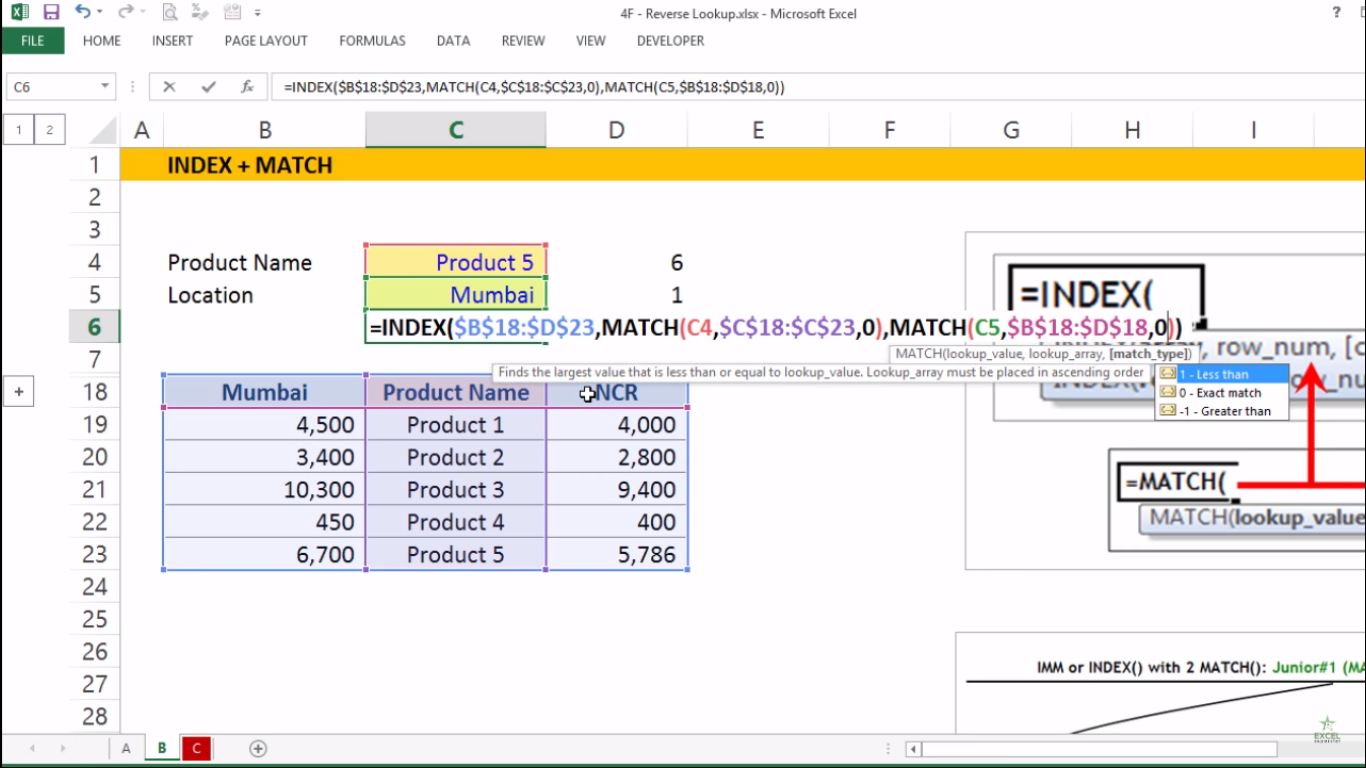The third parameter match_type means 1 =less than 0 = exact match, -1 = greater than

4. Write 0 as exact match and close the bracket =MATCH(C5,\$B\$18:\$D\$18,0)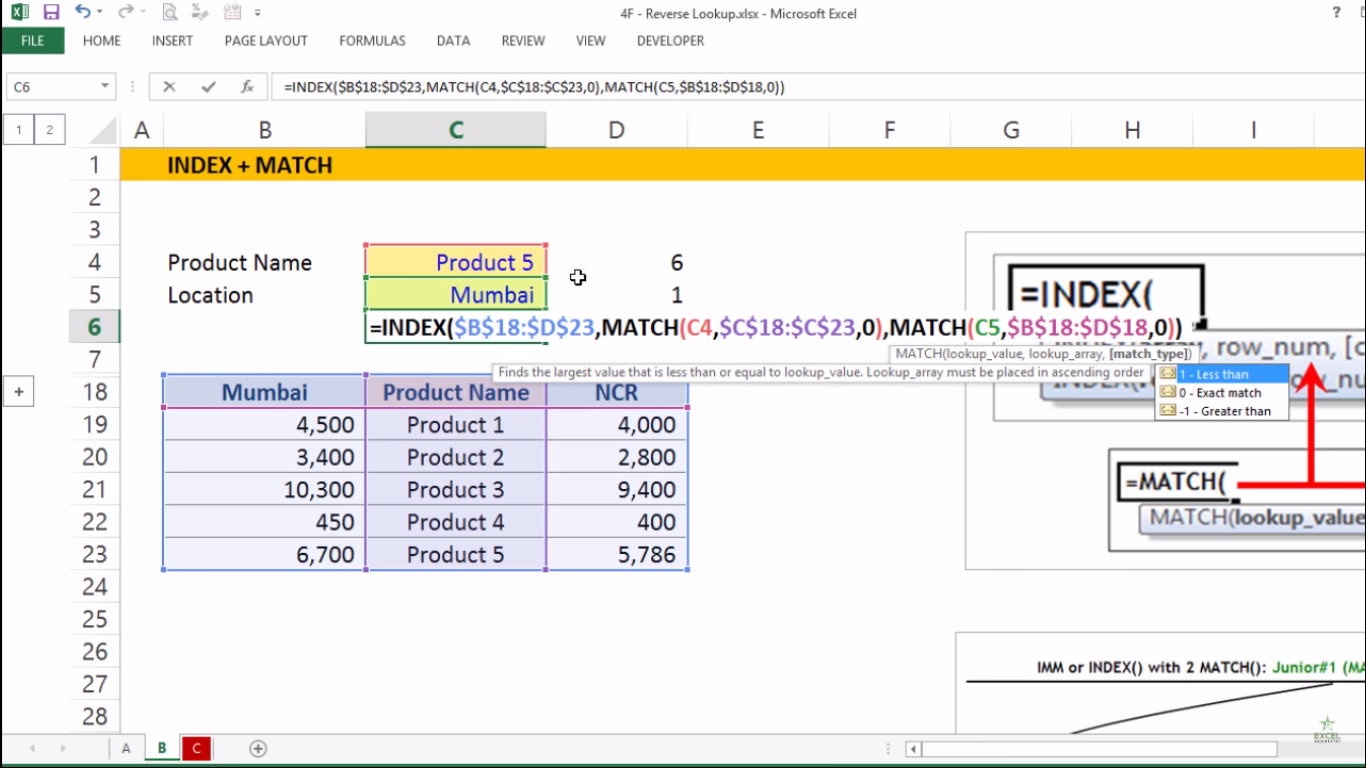5. Lastly, whenever you change the name and location MATCH Formula counts again which helps in input of INDEX Formula